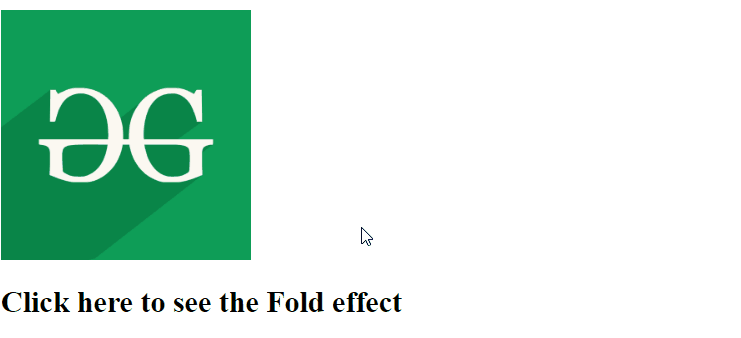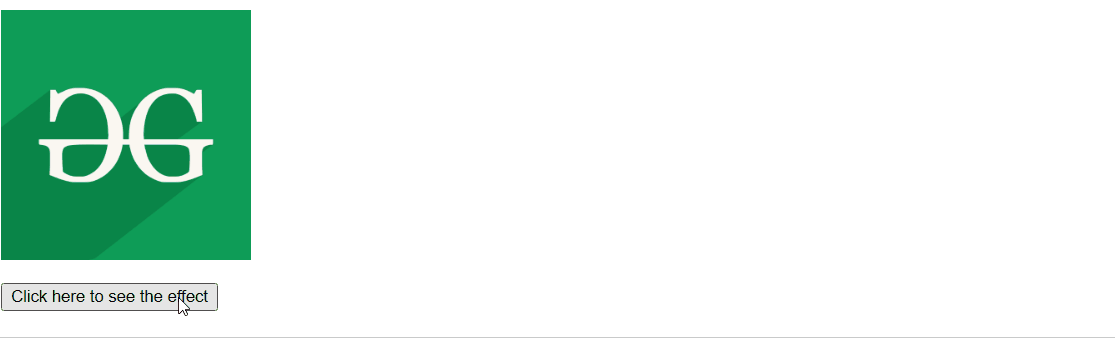# script.aculo.us Fold Effect

• Last Updated : 03 Dec, 2020

In this article, we will demonstrate the effect of Fold by using a JavaScript library called script.aculo.us having a smooth scaling down first in the vertical direction and then horizontal toward the left to finally disappear. We can adjust the duration of the effect as well.

Syntax:

Hey geek! The constant emerging technologies in the world of web development always keeps the excitement for this subject through the roof. But before you tackle the big projects, we suggest you start by learning the basics. Kickstart your web development journey by learning JS concepts with our JavaScript Course. Now at it's lowest price ever!

```Effect.Fold('id_of_element');

// Or

Effect.Fold('id_of_element', { duration: dur });```

Parameters:

• id_of_element: Element on which the effect to be applied.
• duration: Duration taken by this effect.

Approach:

• To demonstrate the use of this function, we have written a small piece of code. In which we have written a small JavaScript function named FoldEffect() method which uses Fold() method of this library.
• By clicking on Click here to see the Fold effect, you will see the effect clearly.

Example 1: To see the effect first install the library and then open the following program in the local environment.

## HTML

 ```<``html``>``  ` `<``head``>``    ``<``script` `src` `= ``"prototype.js"``>`` ` `    ``<``script` `src` `= ``        ``"scriptaculous.js?load = effects"``>``    ````  ` `    ``<``script` `type` `= ``"text/javascript"``>``        ``function FoldEffect(element){``            ``new Effect.Fold(element, {duration:4});``        ``}``    ``````  ` `<``body``>``    ``<``div` `id` `= ``"myimage"` `onclick` `= ``"FoldEffect(this);"``>`` ` `        ``<``img` `height``=``200px` `width``=``200px` `            ``src` `= ``"gfg.png"` `alt` `= ``"gfg logo"` `/>``             ` `        ``<``h2``>Click here to see the Fold effect ``    ``    ````  ` ``

Output:Example 2: In this example, we have changed the duration of the effect and also added a button to see the effect.

## HTML

 ```<``html``>``  ` `<``head``>``    ``<``script` `src` `= ``"prototype.js"``>`` ` `    ``<``script` `src` `= ``        ``"scriptaculous.js?load = effects"``>``    ````  ` `    ``<``script` `type` `= ``"text/javascript"``>``        ``function FoldEffect(element){``        ``new Effect.Fold(element, {duration:3});``        ``}``    ``````  ` `<``body``>``    ``<``div` `id` `= ``"myimage"``>``        ``<``img` `height``=``200px` `width``=``200px` `            ``src` `= ``"gfg.png"` `alt` `= ``"gfg logo"` `/>`` ` `        ``<``br``><``br``>``         ` `        ``<``button` `onclick` `= ``"FoldEffect('myimage');"``>``            ``Click here to see the effect``        ````    ``    ````  ` ``

Output:My Personal Notes arrow_drop_up